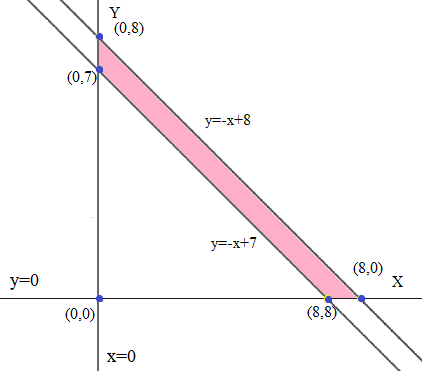# Evaluate the integral by making an appropriate change of variables. Let \iint_R 3...

## Question:

Evaluate the integral by making an appropriate change of variables.

Let {eq}\iint_R 3 \cos\Big(3\Big(y - \frac{x}{y} + x\Big)\Big) dA {/eq} where R is the trapezoidal region with vertices {eq}(7, 0) {/eq}, {eq}(8, 0) {/eq}, {eq}(0, 8) {/eq}, and {eq}(0, 7) {/eq}.

## Transformations To Solve Double Integrals:

If a function F(x,y) is to be integrated over a region R bounded by some given curves in XY coordinate system, then the integration can be simplified under som transformation {eq}\displaystyle x=g(u,v) {/eq} and {eq}\displaystyle y=h(u,v) {/eq} which transform region R to some region Q such that the integral is transformed to some simpler integral given by,

$$\displaystyle \iint_RF(x,y) \ dA=\iint_QF(g(u,v),h(u,v))\left|\frac{\partial(x,y)}{\partial(u,v)}\right| \ d\bar{A}$$

where,{eq}\displaystyle \left|\frac{\partial(x,y)}{\partial(u,v)}\right|=\frac{\partial x}{\partial u}\frac{\partial y}{\partial v}-\frac{\partial x}{\partial v}\frac{\partial y}{\partial u} \quad \text{ and } \quad \displaystyle d\bar{A}=\ du \ dv {/eq}

To ensure that the transformation simplifies the calculation, the choice of transformation is the key. Else we may end up with an integral which would be even more difficult to handle.

Please refer the figure given belowLet us consider the transformation {eq}\displaystyle u=3(x-y) \text{ and }v=y+x {/eq}. Under this transformation the x and y values in terms of u and v will be given by,

{eq}\displaystyle \Rightarrow x=\frac{u+3v}{6}\text{ and }y=\frac{3v-u}{6} {/eq}

The region R is given bounded by the four given points forming a trapezoid as shown in the above figure, which will have four straight lines with the equations as shown below (under the considered transformations)

{eq}\displaystyle y=-x+7\Rightarrow \frac{3v-u}{6}=-\frac{3v+u}{6}+7\Rightarrow v=7\\ \displaystyle y=-x+8\Rightarrow \frac{3v-u}{6}=-\frac{3v+u}{6}+8\Rightarrow v=8\\ \displaystyle y=0\Rightarrow \frac{3v-u}{6}=0\Rightarrow u=3v\\ \displaystyle x=0\Rightarrow \frac{3v+u}{6}=0\Rightarrow u=-3v\\ {/eq}

Next the Jacobian will be

{eq}\displaystyle \left|\frac{\partial(x,y)}{\partial(u,v)}\right|=\frac{1}{6}\frac{1}{2}-\frac{1}{2}\frac{-1}{6}=\frac{1}{6} {/eq}

So the given integral will be transformed to

{eq}\displaystyle \begin{align} \iint_R3\cos\left( 3\left( \frac{y-x}{y+x}\right)\right) \ dA&=\iint_R3\cos\left( 3\left( \frac{x-y}{y+x}\right)\right) \ dA& \because \cos(-a)=\cos(a)\\ &=\int_{7}^{8}\int_{-3v}^{3v}3\cos\left( \frac{u}{v}\right)\left|\frac{1}{6}\right| \ du \ dv\\ &=\frac{1}{2}\int_{7}^{8}\left[ \sin\left( \frac{u}{v}\right)(v)\right]_{-3v}^{3v} \ du \ dv\\ &=\frac{1}{2}\int_{7}^{8}\left[v(\sin(3))-v\sin(-3)\right] \ dv\\ &=\frac{1}{2}\int_{7}^{8}\left[2v\sin(3)\right] \ dv&\because \sin(-a)=-\sin(a)\\ &=\sin(3)\int_{7}^{8}v \ dv\\ &=\sin(3)\left[\frac{v^2}{2}\right]_{7}^{8}\\ &=\frac{\sin(3)}{2}[64-49]\\ &=\frac{15\sin(3)}{2}\approx 1.0584 \end{align} {/eq}